# 六、SciPy 统计推断

## 6.1 效应量

``````from __future__ import print_function, division

import numpy
import scipy.stats

import matplotlib.pyplot as pyplot

from IPython.html.widgets import interact, fixed
from IPython.html import widgets

# 为随机数生成器播种，所以我们得到相同结果
numpy.random.seed(17)

# 来自 http://colorbrewer2.org/ 的一些漂亮的颜色
COLOR1 = '#7fc97f'
COLOR2 = '#beaed4'
COLOR3 = '#fdc086'
COLOR4 = '#ffff99'
COLOR5 = '#386cb0'

%matplotlib inline
``````

``````mu1, sig1 = 178, 7.7
male_height = scipy.stats.norm(mu1, sig1)

mu2, sig2 = 163, 7.3
female_height = scipy.stats.norm(mu2, sig2)
``````

``````def eval_pdf(rv, num=4):
mean, std = rv.mean(), rv.std()
xs = numpy.linspace(mean - num*std, mean + num*std, 100)
ys = rv.pdf(xs)
return xs, ys
``````

``````xs, ys = eval_pdf(male_height)
pyplot.plot(xs, ys, label='male', linewidth=4, color=COLOR2)

xs, ys = eval_pdf(female_height)
pyplot.plot(xs, ys, label='female', linewidth=4, color=COLOR3)
pyplot.xlabel('height (cm)')
None
``````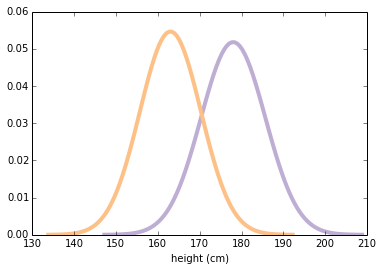``````male_sample = male_height.rvs(1000)

female_sample = female_height.rvs(1000)
``````

``````mean1, std1 = male_sample.mean(), male_sample.std()
mean1, std1

# (178.16511665818112, 7.8419961712899502)
``````

``````mean2, std2 = female_sample.mean(), female_sample.std()
mean2, std2

# (163.48610226651135, 7.382384919896662)
``````

Now, there are many ways to describe the magnitude of the difference between these distributions. An obvious one is the difference in the means:

``````difference_in_means = male_sample.mean() - female_sample.mean()
difference_in_means # 单位为厘米

# 14.679014391669767
``````

• 如果不了解更多关于分布的信息（比如标准差），很难解释 15 厘米之间的差异是否很大。

• 差异的大小取决于度量单位，因此很难在不同的研究中进行比较。

``````# 练习：均值的相对差异，表示成百分比是什么？

relative_difference = difference_in_means / male_sample.mean()
relative_difference * 100   # 百分比
# 8.2389946286916569
``````

``````relative_difference = difference_in_means / female_sample.mean()
relative_difference * 100    # 百分比
# 8.9787536605040401
``````

### 第二部分

``````simple_thresh = (mean1 + mean2) / 2
simple_thresh

# 170.82560946234622
``````

``````thresh = (std1 * mean2 + std2 * mean1) / (std1 + std2)
thresh

# 170.6040359174722
``````

``````male_below_thresh = sum(male_sample < thresh)
male_below_thresh

# 164
``````

``````female_above_thresh = sum(female_sample > thresh)
female_above_thresh

# 174
``````

“重叠”是曲线下面的总面积，最终位于阈值的右侧。

``````overlap = male_below_thresh / len(male_sample) + female_above_thresh / len(female_sample)
overlap

# 0.33799999999999997
``````

``````misclassification_rate = overlap / 2
misclassification_rate

# 0.16899999999999998
``````

``````# 练习：假设我随机选择男性和女性。
# 男性更高的概率是什么？
sum(x > y for x, y in zip(male_sample, female_sample)) / len(male_sample)

# 0.91100000000000003
``````

• 作为概率，它们不依赖于度量单位，因此它们在研究之间具有可比性。

• 它们以操作术语表达，因此读者可以了解差异所产生的实际效果。

``````def CohenEffectSize(group1, group2):
"""计算 Cohen d。

group1: Series 或 NumPy 数组
group2: Series 或 NumPy 数组

返回值: float
"""
diff = group1.mean() - group2.mean()

n1, n2 = len(group1), len(group2)
var1 = group1.var()
var2 = group2.var()

pooled_var = (n1 * var1 + n2 * var2) / (n1 + n2)
d = diff / numpy.sqrt(pooled_var)
return d
``````

``````CohenEffectSize(male_sample, female_sample)
``````

1.9274780043619493

``````def overlap_superiority(control, treatment, n=1000):
"""基于样本估计重叠和有时。

control: scipy.stats rv 对象
treatment: scipy.stats rv 对象
n: 样本大小
"""
control_sample = control.rvs(n)
treatment_sample = treatment.rvs(n)
thresh = (control.mean() + treatment.mean()) / 2

control_above = sum(control_sample > thresh)
treatment_below = sum(treatment_sample < thresh)
overlap = (control_above + treatment_below) / n

superiority = sum(x > y for x, y in zip(treatment_sample, control_sample)) / n
return overlap, superiority
``````

``````def plot_pdfs(cohen_d=2):
"""为标准差不同的分布绘制 PDF。

cohen_d: 均值之间的标准差
"""
control = scipy.stats.norm(0, 1)
treatment = scipy.stats.norm(cohen_d, 1)
xs, ys = eval_pdf(control)
pyplot.fill_between(xs, ys, label='control', color=COLOR3, alpha=0.7)

xs, ys = eval_pdf(treatment)
pyplot.fill_between(xs, ys, label='treatment', color=COLOR2, alpha=0.7)

o, s = overlap_superiority(control, treatment)
print('overlap', o)
print('superiority', s)
``````

``````plot_pdfs(2)

'''
overlap 0.278
superiority 0.932
'''
````````````slider = widgets.FloatSliderWidget(min=0, max=4, value=2)
interact(plot_pdfs, cohen_d=slider)
None

'''
overlap 0.305
superiority 0.931
'''
``````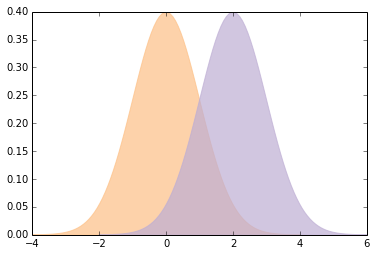Cohen 的`d`有一些不错的属性：

• 因为平均值和标准差具有相同的单位，它们的比例是无量纲的，所以我们可以比较不同研究中的`d`

• 在通常使用`d`的字段中，人们会进行校准，来了解哪些值应该被认为是大的，令人惊讶的或重要的。

• 给定`d`（并假设分布是正态），你可以计算重叠，优势和相关统计量。

## 6.2 随机采样

``````from __future__ import print_function, division

import numpy
import scipy.stats

import matplotlib.pyplot as pyplot

from IPython.html.widgets import interact, fixed
from IPython.html import widgets

# 为随机数生成器播种，所以我们得到相同结果
numpy.random.seed(18)

# 来自 http://colorbrewer2.org/ 一些漂亮的颜色
COLOR1 = '#7fc97f'
COLOR2 = '#beaed4'
COLOR3 = '#fdc086'
COLOR4 = '#ffff99'
COLOR5 = '#386cb0'

%matplotlib inline
``````

### 第一部分

``````weight = scipy.stats.lognorm(0.23, 0, 70.8)
weight.mean(), weight.std()

# (72.697645732966876, 16.944043048498038)
``````

``````xs = numpy.linspace(20, 160, 100)
ys = weight.pdf(xs)
pyplot.plot(xs, ys, linewidth=4, color=COLOR1)
pyplot.xlabel('weight (kg)')
pyplot.ylabel('PDF')
None
``````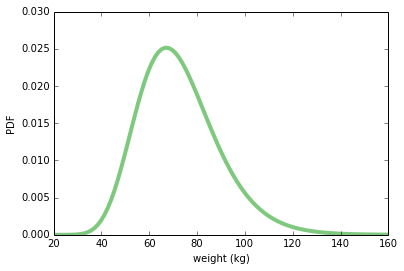`make_sample`从此分布中抽取随机样本。 结果是 NumPy 数组。

``````def make_sample(n=100):
sample = weight.rvs(n)
return sample
``````

``````sample = make_sample(n=100)
sample.mean(), sample.std()

# (76.308293640077437, 19.995558735561865)
``````

``````def sample_stat(sample):
return sample.mean()
``````

“实验”的一次迭代是收集 100 名女性的样本并计算他们的平均体重。我们可以模拟多次运行此实验，并收集样本统计量的列表。 结果是NumPy数组。

``````def compute_sample_statistics(n=100, iters=1000):
stats = [sample_stat(make_sample(n)) for i in range(iters)]
return numpy.array(stats)
``````

``````sample_means = compute_sample_statistics(n=100, iters=1000)
``````

``````pyplot.hist(sample_means, color=COLOR5)
pyplot.xlabel('sample mean (n=100)')
pyplot.ylabel('count')
None
``````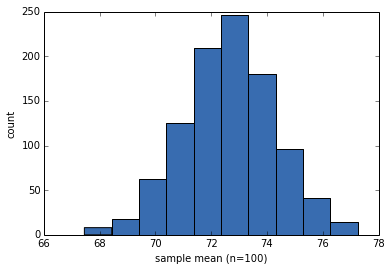``````sample_means.mean()

# 72.652052080657413
``````

``````std_err = sample_means.std()
std_err

# 1.6355262477017491
``````

``````conf_int = numpy.percentile(sample_means, [5, 95])
conf_int

# array([ 69.92149384,  75.40866638])
``````

``````def summarize_sampling_distribution(sample_stats):
print('SE', sample_stats.std())
print('90% CI', numpy.percentile(sample_stats, [5, 95]))
``````

``````summarize_sampling_distribution(sample_means)

'''
SE 1.6355262477
90% CI [ 69.92149384  75.40866638]
'''
``````

``````def plot_sample_stats(n, xlim=None):
sample_stats = compute_sample_statistics(n, iters=1000)
summarize_sampling_distribution(sample_stats)
pyplot.hist(sample_stats, color=COLOR2)
pyplot.xlabel('sample statistic')
pyplot.xlim(xlim)
``````

``````plot_sample_stats(100)

'''
SE 1.71202891175
90% CI [ 69.96057332  75.58582662]
'''
``````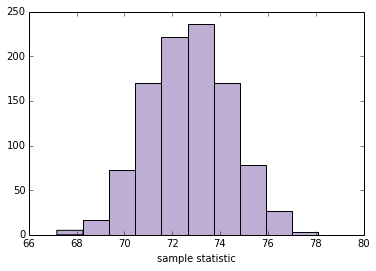``````def sample_stat(sample):
return sample.mean()

slider = widgets.IntSliderWidget(min=10, max=1000, value=100)
interact(plot_sample_stats, n=slider, xlim=fixed([55, 95]))
None

'''
SE 1.71314776815
90% CI [ 69.99274896  75.65943185]
'''
``````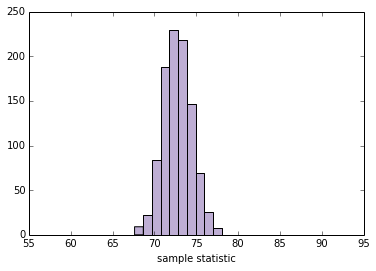• 样本标准差
• 变异系数，即样本标准差除以样本标准均值。
• 最小值或最大值
• 中位数（第 50 个百分位数）
• 第 10 或 90 个百分位数
• 四分位数间距（IQR），即第 75 和第 25 百分位数之间的差。

``````def sample_stat(sample):
# TODO：将下面一行替换为其它样本统计量
return sample.mean()

slider = widgets.IntSliderWidget(min=10, max=1000, value=100)
interact(plot_sample_stats, n=slider, xlim=fixed([0, 100]))
None

'''
SE 1.67195986148
90% CI [ 69.82954731  75.32184298]
'''
``````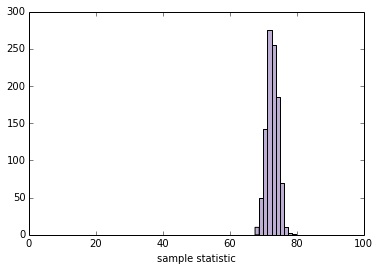### 第二部分

``````class Resampler(object):
"""表示计算采样分布的框架。"""

def __init__(self, sample, xlim=None):
"""储存实际样本。"""
self.sample = sample
self.n = len(sample)
self.xlim = xlim

def resample(self):
"""通过带放回地选择原始样本生成新样本。
"""
new_sample = numpy.random.choice(self.sample, self.n, replace=True)
return new_sample

def sample_stat(self, sample):
"""计算样本统计量，使用原始样本或者模拟样本。
"""
return sample.mean()

def compute_sample_statistics(self, iters=1000):
"""模拟许多实验并收集所得样本统计量。
"""
stats = [self.sample_stat(self.resample()) for i in range(iters)]
return numpy.array(stats)

def plot_sample_stats(self):
"""运行模拟的实验，并汇总结果。
"""
sample_stats = self.compute_sample_statistics()
summarize_sampling_distribution(sample_stats)
pyplot.hist(sample_stats, color=COLOR2)
pyplot.xlabel('sample statistic')
pyplot.xlim(self.xlim)
``````

``````def plot_resampled_stats(n=100):
sample = weight.rvs(n)
resampler = Resampler(sample, xlim=[55, 95])
resampler.plot_sample_stats()
``````

``````plot_resampled_stats(100)

'''
SE 1.72606450921
90% CI [ 71.35648645  76.82647135]
'''
``````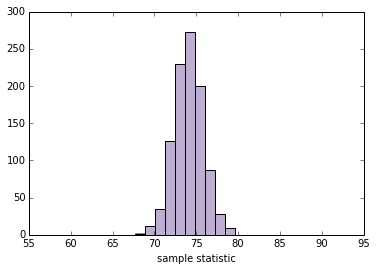``````slider = widgets.IntSliderWidget(min=10, max=1000, value=100)
interact(plot_resampled_stats, n=slider, xlim=fixed([1, 15]))
None

'''
SE 1.67407589545
90% CI [ 69.60129748  75.13161693]
'''
``````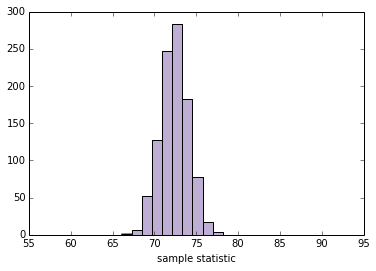``````class StdResampler(Resampler):
"""计算标准差的采样分布。"""

def sample_stat(self, sample):
"""计算样本统计量，使用原始样本或者模拟样本。
"""
return sample.std()
``````

``````def plot_resampled_stats(n=100):
sample = weight.rvs(n)
resampler = StdResampler(sample, xlim=[0, 100])
resampler.plot_sample_stats()

plot_resampled_stats()

'''
SE 1.30056137605
90% CI [ 13.70615766  18.05008376]
'''
````````````slider = widgets.IntSliderWidget(min=10, max=1000, value=100)
interact(plot_resampled_stats, n=slider)
None

'''
SE 1.29095098626
90% CI [ 15.13442137  19.27452588]
'''
``````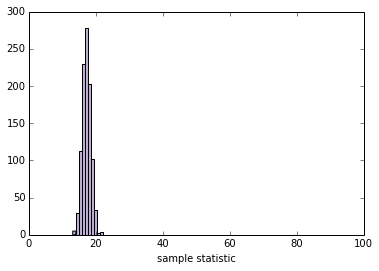### 第三部分

``````female_weight = scipy.stats.lognorm(0.23, 0, 70.8)
female_weight.mean(), female_weight.std()

# (72.697645732966876, 16.944043048498038)
``````

``````male_weight = scipy.stats.lognorm(0.20, 0, 87.3)
male_weight.mean(), male_weight.std()

# (89.063576984335782, 17.992335889366288)
``````

``````female_sample = female_weight.rvs(100)
male_sample = male_weight.rvs(100)
``````

``````male_sample.mean() - female_sample.mean()

# 15.521337537414979
``````

``````def CohenEffectSize(group1, group2):
"""计算 Cohen d。

group1: Series 或 NumPy 数组
group2: Series 或 NumPy 数组

返回值: float
"""
diff = group1.mean() - group2.mean()

n1, n2 = len(group1), len(group2)
var1 = group1.var()
var2 = group2.var()

pooled_var = (n1 * var1 + n2 * var2) / (n1 + n2)
d = diff / numpy.sqrt(pooled_var)
return d
``````

``````CohenEffectSize(male_sample, female_sample)

# 0.9271315108152719
``````

``````class CohenResampler(Resampler):
def __init__(self, group1, group2, xlim=None):
self.group1 = group1
self.group2 = group2
self.xlim = xlim

def resample(self):
group1 = numpy.random.choice(self.group1, len(self.group1), replace=True)
group2 = numpy.random.choice(self.group2, len(self.group2), replace=True)
return group1, group2

def sample_stat(self, groups):
group1, group2 = groups
return CohenEffectSize(group1, group2)

# 注：下面的函数和 Resampler 中相同，
# 所以我可以仅仅继承它，但是我为了可读性而包含它
def compute_sample_statistics(self, iters=1000):
stats = [self.sample_stat(self.resample()) for i in range(iters)]
return numpy.array(stats)

def plot_sample_stats(self):
sample_stats = self.compute_sample_statistics()
summarize_sampling_distribution(sample_stats)
pyplot.hist(sample_stats, color=COLOR2)
pyplot.xlabel('sample statistic')
pyplot.xlim(self.xlim)
``````

``````resampler = CohenResampler(male_sample, female_sample)
resampler.plot_sample_stats()

'''
SE 0.160707033098
90% CI [ 0.6808076  1.1974013]
'''
``````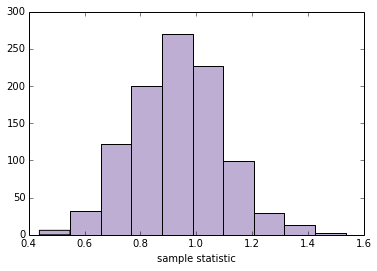## 6.3 假设检验

``````from __future__ import print_function, division

import numpy
import scipy.stats

import matplotlib.pyplot as pyplot

from IPython.html.widgets import interact, fixed
from IPython.html import widgets

import first

# 为随机数生成器播种，所以我们得到相同结果
numpy.random.seed(19)

# 来自 http://colorbrewer2.org/ 的一些漂亮的颜色
COLOR1 = '#7fc97f'
COLOR2 = '#beaed4'
COLOR3 = '#fdc086'
COLOR4 = '#ffff99'
COLOR5 = '#386cb0'

%matplotlib inline
``````

### 第一部分

``````live, firsts, others = first.MakeFrames()
``````

``````def TestStatistic(data):
group1, group2 = data
test_stat = abs(group1.mean() - group2.mean())
return test_stat
``````

``````group1 = firsts.prglngth
group2 = others.prglngth
``````

``````actual = TestStatistic((group1, group2))
actual

# 0.078037266777549519
``````

``````n, m = len(group1), len(group2)
pool = numpy.hstack((group1, group2))
``````

``````def RunModel():
numpy.random.shuffle(pool)
data = pool[:n], pool[n:]
return data
``````

``````RunModel()

# (array([36, 40, 39, ..., 43, 42, 40]), array([43, 39, 32, ..., 37, 35, 41]))
``````

``````TestStatistic(RunModel())

# 0.081758440969863955
``````

``````test_stats = numpy.array([TestStatistic(RunModel()) for i in range(1000)])
test_stats.shape

# (1000,)
``````

``````def VertLine(x):
"""在 x 处绘制竖直线。"""
pyplot.plot([x, x], [0, 300], linewidth=3, color='0.8')

VertLine(actual)
pyplot.hist(test_stats, color=COLOR5)
pyplot.xlabel('difference in means')
pyplot.ylabel('count')
None
``````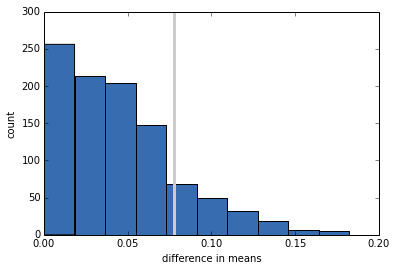p 值是零假设下的检验统计量超过实际值的概率。

``````pvalue = sum(test_stats >= actual) / len(test_stats)
pvalue

# 0.14999999999999999
``````

### 第二部分

``````class HypothesisTest(object):
"""表示假设检验。"""

def __init__(self, data):
"""初始化。

data: 任何格式相关的数据
"""
self.data = data
self.MakeModel()
self.actual = self.TestStatistic(data)
self.test_stats = None

def PValue(self, iters=1000):
"""计算测试统计量的分布和 p 值。

iters: 迭代数

返回值: float p 值
"""
self.test_stats = numpy.array([self.TestStatistic(self.RunModel())
for _ in range(iters)])

count = sum(self.test_stats >= self.actual)
return count / iters

def MaxTestStat(self):
"""返回模拟期间见到的最大的测试统计量。
"""
return max(self.test_stats)

def PlotHist(self, label=None):
"""使用观测测试统计量处的竖直线条绘制 Cdf。
"""
def VertLine(x):
"""在 x 处绘制竖直线条。"""
pyplot.plot([x, x], [0, max(ys)], linewidth=3, color='0.8')

ys, xs, patches = pyplot.hist(ht.test_stats, color=COLOR4)
VertLine(self.actual)
pyplot.xlabel('test statistic')
pyplot.ylabel('count')

def TestStatistic(self, data):
"""计算测试统计量。

data: 任何格式相关的数据
"""
raise UnimplementedMethodException()

def MakeModel(self):
"""为零假设构建模型
"""
pass

def RunModel(self):
"""运行零假设的模型。

返回值: 模拟的数据
"""
raise UnimplementedMethodException()
``````

`HypothesisTest`是一个对模板进行编码的抽象父类。子类填写缺少的方法。 例如，这是上一节的测试。

``````class DiffMeansPermute(HypothesisTest):
"""通过置换测试均值差异。"""

def TestStatistic(self, data):
"""计算测试统计量.

data: 任何格式相关的数据
"""
group1, group2 = data
test_stat = abs(group1.mean() - group2.mean())
return test_stat

def MakeModel(self):
"""构建零假设的模型。
"""
group1, group2 = self.data
self.n, self.m = len(group1), len(group2)
self.pool = numpy.hstack((group1, group2))

def RunModel(self):
"""运行零假设的模型。

返回值: 模拟的数据
"""
numpy.random.shuffle(self.pool)
data = self.pool[:self.n], self.pool[self.n:]
return data
``````

``````data = (firsts.prglngth, others.prglngth)
ht = DiffMeansPermute(data)
p_value = ht.PValue(iters=1000)
print('\nmeans permute pregnancy length')
print('p-value =', p_value)
print('actual =', ht.actual)
print('ts max =', ht.MaxTestStat())
``````

means permute pregnancy length p-value = 0.16 actual = 0.0780372667775 ts max = 0.173695697482

``````ht.PlotHist()
``````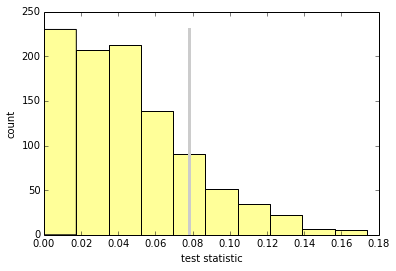``````class DiffStdPermute(DiffMeansPermute):
"""通过置换测试均值差异。"""

def TestStatistic(self, data):
"""计算测试统计量。

data: 任何格式相关的数据
"""
group1, group2 = data
test_stat = abs(group1.std() - group2.std())
return test_stat

data = (firsts.prglngth, others.prglngth)
ht = DiffStdPermute(data)
p_value = ht.PValue(iters=1000)
print('\nstd permute pregnancy length')
print('p-value =', p_value)
print('actual =', ht.actual)
print('ts max =', ht.MaxTestStat())

'''
std permute pregnancy length
p-value = 0.155
actual = 0.176049064229
ts max = 0.44299505029
'''
``````

``````data = (firsts.totalwgt_lb.dropna(), others.totalwgt_lb.dropna())
ht = DiffMeansPermute(data)
p_value = ht.PValue(iters=1000)
print('\nmeans permute birthweight')
print('p-value =', p_value)
print('actual =', ht.actual)
print('ts max =', ht.MaxTestStat())

'''
means permute birthweight
p-value = 0.0
actual = 0.124761184535
ts max = 0.0917504268392
'''
``````